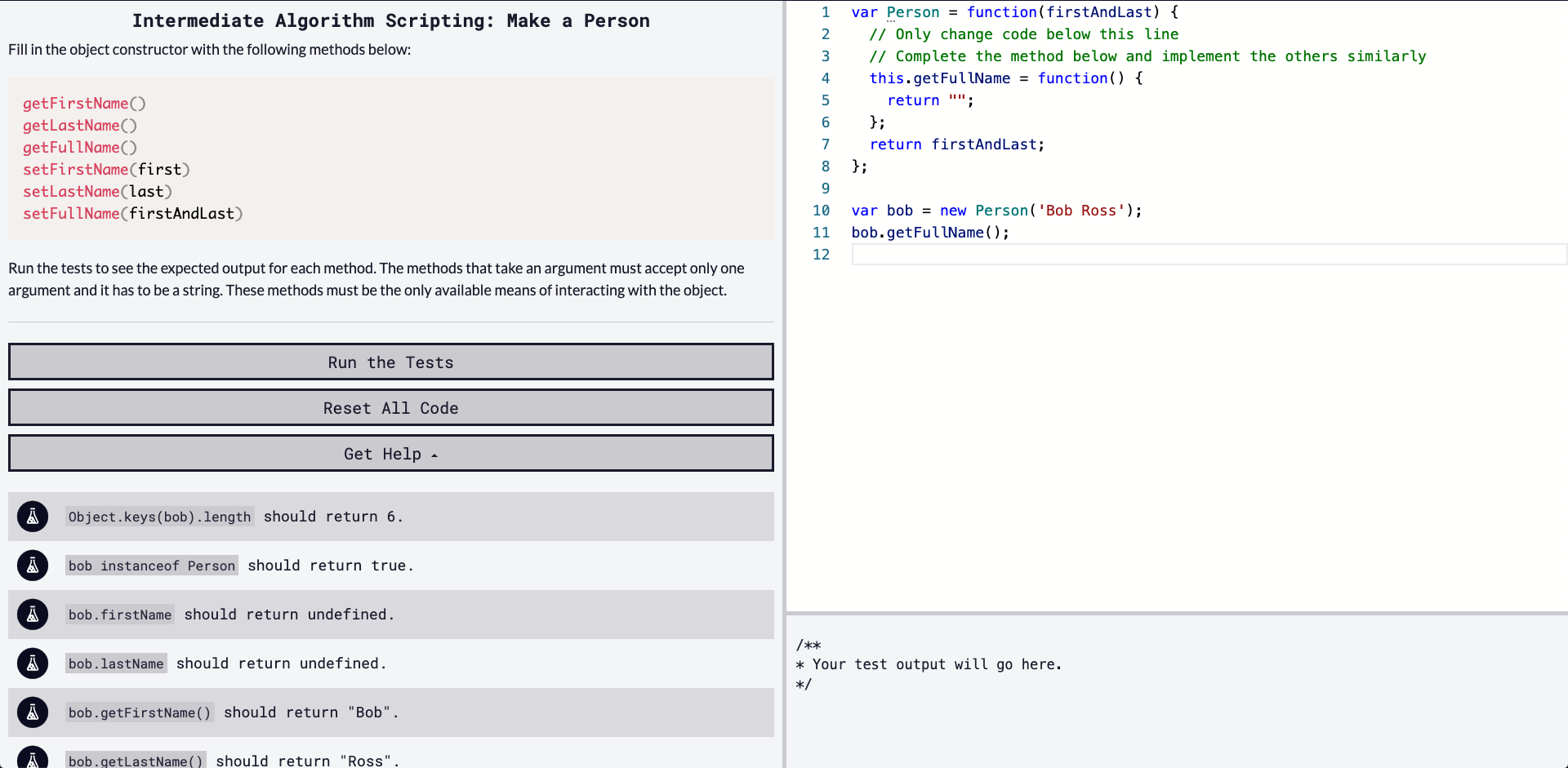## DEV Community

Viren B

Posted on • Originally published at virenb.cc

# Solving "Make a Person" / freeCodeCamp Algorithm Challenges

** Post can also be found on virenb.cc **Let's solve freeCodeCamp's intermediate algorithm scripting challenge, 'Make a Person'.

### Starter Code

``````var Person = function(firstAndLast) {
// Only change code below this line
// Complete the method below and implement the others similarly
this.getFullName = function() {
return "";
};
return firstAndLast;
};

var bob = new Person('Bob Ross');
bob.getFullName();
``````

### Instructions

Fill in the object constructor with the following methods below:

``````getFirstName()
getLastName()
getFullName()
setFirstName(first)
setLastName(last)
setFullName(firstAndLast)
``````

Run the tests to see the expected output for each method. The methods that take an argument must accept only one argument and it has to be a string. These methods must be the only available means of interacting with the object.

### Test Cases

• `Object.keys(bob).length` should return 6.
• `bob instanceof Person` should return true.
• `bob.firstName` should return undefined.
• `bob.lastName` should return undefined.
• `bob.getFirstName()` should return "Bob".
• `bob.getLastName()` should return "Ross".
• `bob.getFullName()` should return "Bob Ross".
• `bob.getFullName()` should return "Haskell Ross" after `bob.setFirstName("Haskell")`.
• `bob.getFullName()` should return "Haskell Curry" after `bob.setLastName("Curry")`.
• `bob.getFullName()` should return "Haskell Curry" after `bob.setFullName("Haskell Curry")`.
• `bob.getFirstName()` should return "Haskell" after `bob.setFullName("Haskell Curry")`.
• `bob.getLastName()` should return "Curry" after `bob.setFullName("Haskell Curry")`.

# Our Approach

The instructions for this challenge are short and to the point.

• We're working with a function expression, with an argument of `firstAndLast`.
• It is an object constructor that we need to complete. The instructions mention methods which must be included in the object constructor.

This challenge is a little unique as we have to build out and complete an object constructor. freeCodeCamp provided a few lessons about object constructors from what I remember so it may be helpful to go back and look at them.

The instructions let us know which methods have to be created, so let's get down to it.

The argument for this constructor is `firstAndLast`, which will be a string of a first and last name, separated by a white space (according to our test cases). I think it will be handy to separate out the first and last name into their own variables as some methods require us to return either name. We can deconstruct the argument as seen below.

``````let [firstName, lastName] = firstAndLast.split(' ');
// We're splitting on the white space between the two names

var Person = function(firstAndLast) {
let [firstName, lastName] = firstAndLast.split(' ');
console.log(firstName, lastName)
};

Person('Bob World') // Bob, World
``````

Okay, we won't need the `console.log()` in our solution but we're off to a good start.

Before we dive into approaching each method, I found this to be good documentation about constructor functions.

``````getFirstName()
``````

This method, as it states, will return the first name. We have that in a variable already. So our code would look something like this:

``````this.getFirstName = function() {
return firstName;
}
``````

Our next method will be `getLastName()` will be the pretty much the same.

``````this.getLastName = function() {
return lastName;
}
``````

Let's skip over `getFullName()` for now. The reason is it will have to be updated if we run a 'set' method to change the name.

For `setFirstName()`, we'll have it take in an argument, and then update the `firstName` variable to the new one provided in this function.

``````this.setFirstName = function (first) {
firstName = first;
return firstName;
}
``````

`setLastName()` will work very similarly.

``````this.setLastName = function (last) {
lastName = last;
return lastName;
}
``````

The most challenging method is `setFullName()`. We will have to replace both variables with the new argument provided in this function. Like the argument of the constructor function, we assume (based off the test cases), it will be a string with a first and last name, separated by a white space.

``````this.setFullName = function (firstAndLast) {
firstName = firstAndLast.split(' ');
lastName = firstAndLast.split(' ');
return `\${firstName} \${lastName}`;
}
``````

MDN: String.split()

We take the argument, split it at the white space, and then set each string into the firstName and lastName variables.

Returning to the last method we didnt cover, `getFullName()`.

``````this.getFullName = function () {
return `\${firstName} \${lastName}`;
};
``````

# Our Solution

``````var Person = function(firstAndLast) {
// Complete the method below and implement the others similarly

let [firstName, lastName] = firstAndLast.split(' ');

this.getFirstName = function() {
return firstName;
};

this.getLastName = function() {
return lastName;
};

this.getFullName = function() {
return `\${firstName} \${lastName}`;
};

this.setFirstName = function(first) {
firstName = first;
return firstName;
};

this.setLastName = function(last) {
lastName = last;
return lastName;
}

this.setFullName = function(firstAndLast) {
firstName = firstAndLast.split(' ');
lastName = firstAndLast.split(' ');
return `\${firstName} \${lastName}`;
}

};
``````

'Make a Person' Challenge on fCC

freeCodeCamp

Donate to FCC!

Solution on my GitHub

DEV Community## 11 Tips That Make You a Better Typescript Programmer

### 1 Think in {Set}

Type is an everyday concept to programmers, but it’s surprisingly difficult to define it succinctly. I find it helpful to use Set as a conceptual model instead.

### #2 Understand declared type and narrowed type

One extremely powerful typescript feature is automatic type narrowing based on control flow. This means a variable has two types associated with it at any specific point of code location: a declaration type and a narrowed type.

...